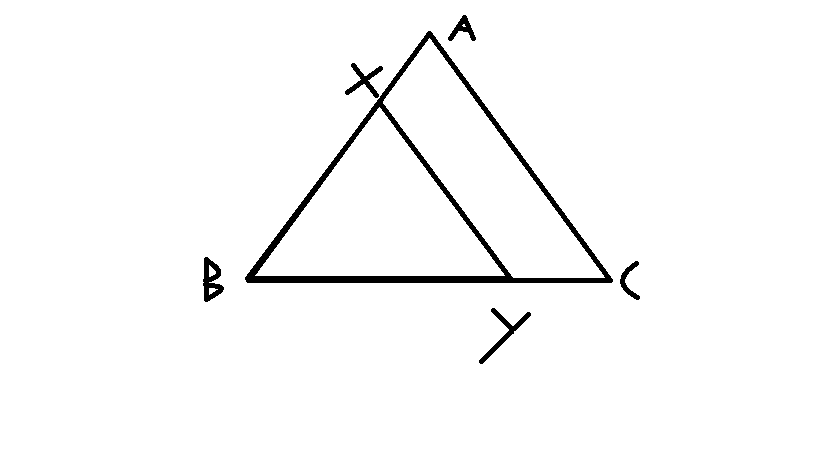# Don't be greedy

Geometry Level 3In figure above, the line segment $XY$ is parallel to side $AC$ of triangle $ABC$ into two parts of equal areas.

Find the ratio $AX:AB$.

×